ET online
September 2016
Turns Ratio Testing: Hand crank versus automatic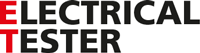# Turns Ratio Testing: Hand crank versus automatic

01 September 2016

Jill Duplessis - Global technical marketing manager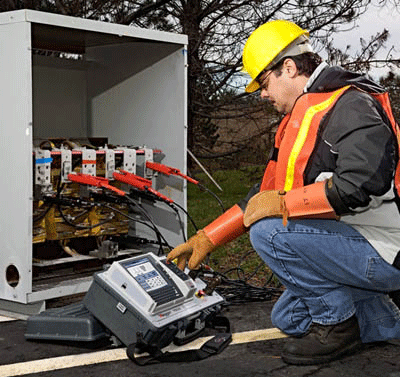A transformer turns ratio test provides a quick verification of the most fundamental operational characteristic of a transformer – its ability to transform voltage as anticipated. In doing so, the test provides invaluable reassurance to the operator. Open- and short-circuit conditions in transformer main and tap windings may cause the transformer turns ratio to change and therefore this test is at once providing useful diagnostic information.

Engineers have noted, however, that traditional “hand-crank” TTR instruments sometimes give values for turns ratio that are different from those given by modern automated TTR instruments. Indeed, many insist that the traditional instruments provide results that are more dependable. But is this actually true? And why do the instruments produce different results?

Background

To answer these questions, let’s start by considering an ideal transformer. For such a transformer, the ratio of the terminal voltages (that is, the field-measured no-load voltage ratio, V1/V2) equals the true ratio of the number of turns on each winding, N1/N2 – the ratio of transformation. This is not true with a real transformer. Here’s why.

The turns ratio is equal to the ratio of the voltages induced by the resultant mutual flux (E1/E2) when a transformer is energized. The voltage induced in a single turn is the same irrespective of whether it is part of the primary or part of the secondary winding, so the total voltage induced in each of the windings by the common flux must be proportional to the number of turns. That is E1/E2 = N1/N2. But in a real transformer, E1, the primary induced voltage, does not exactly equal the applied voltage, V1. In fact, V1 is equal to the phasor sum of E1 plus the primary leakage reactance voltage drop due to the exciting current plus the voltage drop due to the resistance of the primary winding multiplied by the exciting current (Fig. 1). The ratio of transformation is therefore only approximately equal to the ratio of the primary and secondary terminal voltages, e.g., the “voltage ratio” as termed in IEEE C57.152-2013.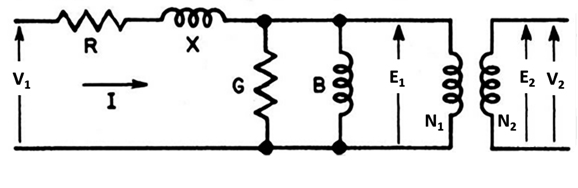Fig. 1: Equivalent circuit for a transformer with no load

In all real transformers, the no-load voltage ratio (measured in the field) is less than the transformation ratio (i.e., the turns ratio). This is because real transformers have losses and magnetic leakage, and they require excitation. Consider, for example, the ratio of primary to secondary currents. Both currents exist together only when the transformer is serving a load so put aside the ratio testing concept (a no-load test) for a moment. Primary current, i1, is the sum of an exciting component, iex, and a load component, iL. The ratio between the secondary current, i2, and the load component, iL, of the primary current is given by:

N1iL = - N2i2 (1)

This can be rewritten as:

N1/N2 = - i2/iL (2)

where the turns ratio = N1/N2, iL = i1 - iex, and iL is always < i1

For an ideal transformer, N1/N2 = i2/i1 (present at the terminals), but for a real transformer, the actual turns ratio (given by equation 2 and which cannot be directly measured) is greater than the ratio of the terminal currents (voltages).

In general, where transformers have large leakage reactance, high magnetising current, high primary resistance or a combination of these, a higher error will be introduced into the measurement of the turns ratio because these factors increase the difference between V1 and E1.

Measuring techniques

Now let’s look at turns ratio measurement techniques. An automated TTR instrument measures the voltage applied to the primary winding terminals and the resulting voltage at the secondary terminals. The instrument calculates the ratio of these voltages and presents this as the transformer turns ratio.

Hand-crank turns ratio instruments work in a different way. The test set is arranged so that the transformer to be tested and the adjustable ratio reference transformer within the test set are connected in parallel and excited from the same voltage source (see Fig. 2). This measurement technique is called a transformer bridge measurement.

Note that the instrument normally excites the transformer under test from its secondary (low voltage) windings. The secondary windings (which, in the case of the transformer under test, is actually the primary winding) are connected in series opposing through a null detector.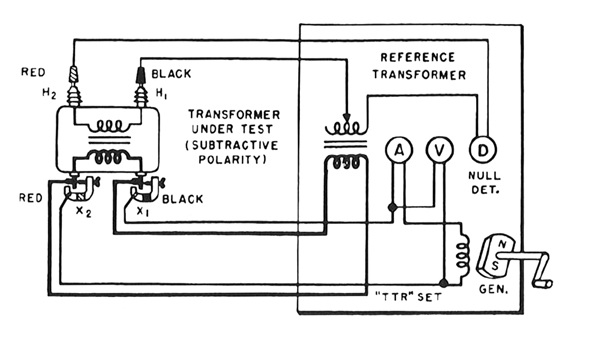Fig. 2

When the ratio of the reference transformer is adjusted so that no current flows in the secondary circuit, as shown by the null detector, two conditions are simultaneously fulfilled. The first is that the voltage ratios of the two transformers are equal and the second is that there is no load on either transformer. The no-load voltage ratio of the reference transformer is known, so the voltage ratio of the transformer under test is kn own, and its turns ratio is therefore also known.

The main source of error with this method is that the primary induced voltage in the transformer under test, EX, and the primary induced voltage in the reference transformer, ET, are likely to be slightly different. Although the same voltage, Vo, (e.g., 8 V) is applied to both transformers, the reference transformer and the transformer under test will have different characteristics, which means that, in general, EX and ET will not be the same. When the reference transformer and the transformer under test both have the same turns ratio – that is, when the null detector senses no current flow – the secondary voltages of the two transformers will also differ by this same percentage error.

Note that the difference between ET and EX, however, will always be less than the difference between ET or EX (whichever is the smaller) and Vo (which represents the error with the automatic method). Also note that in some cases the difference may be small, in which case the error in the measured turns ratio will also be small. In fact, where the transformer under test has the same characteristics as the reference transformer, the error in the turns ratio will be zero. For this reason, TTR reference transformers are designed to match typical distribution and power transformers.

Why choose automated over hand crank?

Transformer manufacturers provide true turns ratio (N1/N2 or E1/E2) on the nameplates of their products and for some transformers, a hand crank TTR will give a result closer to this ratio of transformation than measuring the terminal voltages of the windings. Note, however, that some users claim the hand-crank model provides limited resolution on typical transformer ratios such as 20/1.

If a transformer core is not of an optimum quality, as is sometimes the case, the error in the ratio measured with an automated test set will be greater than the error in a measurement carried out with a hand-crank instrument. In such cases, it is common practice to acknowledge the deficiency of the core, or to revert to the older, hand-crank method. Another approach, provided that the transformer has been operating correctly, is to use the turns ratio measured with an automated TTR as a reference point to which future measurements can be compared.

There are very good reasons why hand crank instruments are now being used less frequently. These include:
1. Their use is considered dangerous because:

a. High voltages up to 1,000 V AC can be produced at instrument terminals. Since the transformer is tested from the LV side, a high voltage is produced on the HV side. If the transformer ratio is greater than 130:1, this voltage will be in excess of 1,000 V.
b. The test leads must be short, which often means that the user of the instrument has to stand on the transformer during testing.

2. Using hand crank TTRs is inefficient because:

a. Only one phase at a time can be tested
b. The instrument requires manual balancing
c. The instrument must be cranked and balanced at the same time
d. Leads must be changed for each phase tested
e. Results have to be recorded manually then transferred manually to the test reports
f. Manual calculation is needed to determine the percentage error
g. Total test time is approximately three to four times longer than with an automatic TTR if the time taken to complete the report is included

3. Hand-crank instruments have limited capability when testing instrument transformers. (They are designed to measure accurately turns ratios of no more than 130/1).

4. Hand-crank instruments excite the transformer under test from the LV winding. Some transformers draw excessive magnetising current and the instrument’s output may be insufficient to excite them from the low voltage winding – for example, network transformers of the 15000/115 V class and some voltage regulators.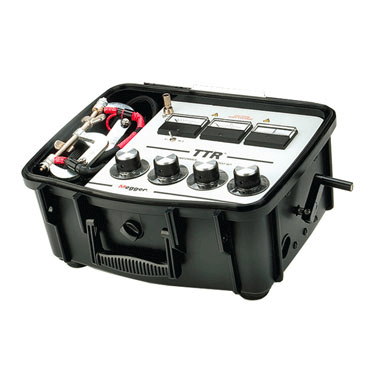Conclusion

There are a few instances where hand crank TTR test sets will give a result that’s closer to the nameplate turns ratio than a modern automated TTR test set. This apparent benefit is, however, more than offset by the many disadvantages of hand crank TTRs, which include time-consuming operation and safety concerns. For these reasons, automated TTRs are replacing their hand-crank counterparts, and this is a trend that will undoubtedly continue.